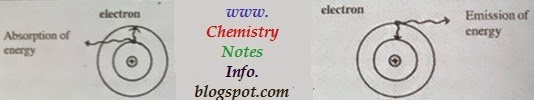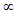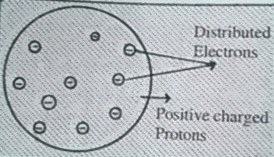## Bohr's Atomic Model

Neils Bohr in 1913 gives a simple model for atomic structure based on the quantum theory.
Main assumption of Bohr atomic model-
1. All atoms consist of dense, very small, positively charged nucleus that have all protons and neutrons in it.
2. Electron revolve around nucleus in definite energy paths known as orbits, shell or energy levels.
3. Orbits denoted by (n). value of n is whole number 1,2,3,4.......... etc. which are represented as K,L,M,N............. etc. respectively.Bohr Atomic Model
4. As we increase the value of n the orbit move farther from nucleus (means distance between nucleus and orbit of higher n is more than smaller n) and their energy also increases so n=1 or K shell have lowest energy.
5. If an electron revolve in same energy level then their is no change in its energy level.
6. As electron absorb energy from outside, it gets exited and move to higher energy level and come back after emitting energy to lower energy level.Absorption and Emission of Electron Energy

## Bohr’s model for hydrogen atom

=>  Explain by nails Bohr (1913).
=>  Postulates for Bohr’s modal are,
1.     Electron in hydrogen atom move around nucleus in circular path of fixed radius and energy. these paths are called orbits
2.     Energy of e does not change with time.
However, when electron move from lower to higher stationary state it absorbed sub amount of energy and energy release when it comes back.
3.     Frequency of radiations emitted or absorbed when transition of e occur is given by
Where, e1 & e2 is lower & higher energy state.
4.     Angular momentum of n electron in given stationary state is given by
[Where n =1,2,3.....]

#### Limitation of Bohr’s model:-

1.     Bohr model fail to explain finer detail of hydrogen atom spectrum observed by spectroscopic, techniques.
2.     It fails to explain spectrum of other atom except hydrogen atom.
3.     It fails to explain splitting of the spectral lines in presence of electric (stark effect) or magnetic field ( Zeeman effect )
4.     Fell to explain formation of molecules from atoms by chemical bonding.

By- Chemistry Notes Info @ www.chemistrynotesinfo.com
Prepared by Jitendra Singh Sandhu
M.Sc. Chemistry

## Rutherford Model of Atom

This experiment is also known as alpa () particle scattering experiment. Rutherford bombard alpha particle on gold foil of thickness 0.0004cm and found that-
1. So many particles passes through the gold foil without any deflection.
2. Some alpha particle are deflected at different angles.
3. Very few about 1 in 20,000 are bounced back (at 360 degree) from gold foil.Rutherford Atomic Model
Rutherford concluded nuclear model of atom as-
1. Most of the part of the atom is hollow and neutral so alpha particles passes straight without any deflection.
2. All positive charge is present in center of atom so alpha particle are deflected at different angles by repulsion from positively charged center known as nucleus.
3. Only very few particles bounced back so size of nucleus is very small as compared to size of atom.
4. Electron moves around nucleus like planet moves around sun in orbits.
5. Mass of electron is negligible so all mass of atom is present in nucleus.
6. Atom is electrically neutral so number of electron is equal to number of proton present in atom.

### Defects of Rutherford Atomic Model

1. Stability of atom is not explained on the basis of this model.
2. This model could not explain discrete spectrum.

### Rutherford’s nuclear model of atom

=>  Given by Rutherford & his students Ernest Marsden and Hans Geiger.
=>  When beam of high energy α- particles was directed at gold foil then tiny flash of light observed at photographic plate.

#### Rutherford observed that-

1)    Most of the α-  practical  passed  through gold foil undeflected :
2)    A small fraction of α- particles was deflected by small angles.
3)    A very few α- particles (about 1 in 20000) bounced back means deflected by nearly 1800

#### From above observations he concludes the structure of atom.

1)    Most of space in atom is empty because most of α- particles passed undeflected.
2)    Few +ve charged α- particles were deflected.
Because + ve charge of the atom present in center in very small volume that repelled & deflected the +ve charged α- particles.
3)    Volume of nucleus is negligible as compared to total volume of atom
i.e.  radius of atom = 10-10m  (approx)
radius of nucleus =  10-15m  (approx)

#### On the basis of observation &  conclusion Rutherford proposed model of atom as-

1)    +ve charge & most of mass present in the center of atom known as nucleus.
2)    Electrons moves around nucleus with very high speed in circular paths known as orbits.
3)    Electrons and nucleus (protons) are held together by electrostatic force of attraction

## Thomson's Atomic ModelThomson's Atomic Model
Thomson's atomic model is first atomic model proposed in 1898 related to atomic structure. This model is similar to water-melon in which positive charge protons is like pulp in which negative charge electrons like seeds in water-melon, also known as pudding model. This model is called Thomson's atomic model.

### Drawback of Thomson's atomic model

Thomson's atomic model is unable to explain scattering experiment of Rutherford.

## Thomson model of atom

=>  Give by J.J Thomson (1898)
=>  According to J.J. Thomson atoms posses a spherical shape with radius about 10-10 m, in which + ve charge is uniformly distributed.
=>  Electrons are embedded in such a manner to give most stable electrostatic arrangement.
=>  Other names of this model plum pudding raisin pudding watermelon.
=>  Mass is assumed to be uniformly distributed in atom.

## Dalton Atomic Theory

John Dalton was an English chemist, physicist, and meteorologist. He developed modern atomic theory.

### Postulates of Dalton's Atomic Theory

The main points of Dalton's atomic theory were explained below-
• Elements are made-up of very small particles known as atoms.
• Atoms of an element are identical in mass, size and many other chemical or physical properties, but atoms of two-different elements differ in mass, size and many other chemical or physical properties.
• Atoms cannot be created, subdivided, or destroyed.
• Chemical compounds are formed, when atoms of different elements (atoms of two or more elements) combine in simple whole number ratios.
• Chemical reactions occurs, when atoms are combined, separated, or rearranged.

### Drawbacks of Dalton's Atomic Theory of Matter

• Atom is indivisible was proved wrong. As an atom can be subdivided into electrons, protons and neutrons. But remember that atom is the tiniest particle that takes part in a chemical reactions.
• According to Dalton Atomic Theory, Atoms of an element are identical in mass, size and many other chemical or physical properties. But practically we observe that atoms of several elements differ in their densities and masses. These atoms with the different masses are known as isotopes. For example, Chlorine (Cl) has 2 isotopes with the mass numbers of 35 and 37.
• Also According to Dalton Atomic Theory, Atoms of two-different elements differ in mass, size and many other chemical or physical properties. But practically we observe that this is not true all times. For example, Argon (Ar) and Calcium (Ca) atoms, as in this case each have an atomic mass of 40 amu. These atoms with similar atomic masses are known as isobars.
• According to Dalton Atomic Theory, Chemical compounds are formed, when atoms of different elements (atoms of two or more elements) combine in simple whole number ratios. But this is not true in case of complex organic compounds.
• Dalton Atomic Theory do not explain the existence of allotropes, means this Dalton atomic theory fails to explain the differences in properties of charcoal, graphite and diamond (allotropes of carbon).

### Supporting Laws for Dalton's Theory

John Dalton based his theory on two laws which are explained below-

#### Law of Conservation of Mass

According to law of conservation of mass, Matter is neither created nor destroyed. Which means in a chemical reaction, amount of elements remains same in starting when only reactants there and at the completion of the reaction when product formed. We always use the “Law of conservation of mass” when we balance chemical equations.

#### Law of Constant Composition

According to law of constant composition, A pure compound will always have the same proportion of the same elements. For example, table salt with molecular formula of NaCl, holds the same proportions of the elements Na (sodium) and Cl (chlorine); no matter from where the salt came from and how much salt one should have.

According to Dalton Atomic theory atom cannot be divided, but after some years it is proved that atom can be divided further by many scientists like J.J.Thomson, L.Rutherford, N.Bohr, Chadwick etc.

### Constituting particles of atom

Atom made up from 3 main particles-
1. Electron 2. Proton 3. NeutronCHEMISTRY NOTES
BY cHEMISTRY nOTES iNFO - wWW.cHEMISTRYnOTESiNFO.cOM